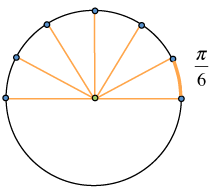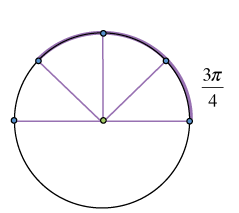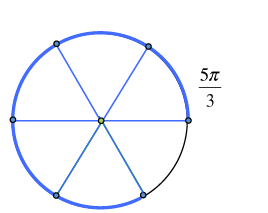### Home > PC3 > Chapter 1 > Lesson 1.3.2 > Problem1-122

1-122.

Sketch a graph of the unit circle with the angles specified below.

1. $\frac { \pi } { 6 }$

Divide the top half of the circle ($\pi$ radians) into six pieces. Select the arc of the first piece.1. $\frac{3\pi}{4}$

Divide the top half of the circle ($\pi$ radians) into four pieces. Select the arc of three of these pieces.1. $\frac{5\pi}{3}$

Divide the top and bottom half of the circle into three pieces each. Select the arc of five of these pieces.